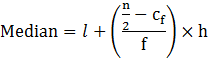Guru

# The lengths of 40 leaves in a plant are measured correctly to the nearest millimeter, and the data obtained is represented as in the following table: Q.4

• 0

How i solve this problem form exercise 14.3 of statistics chapter of question no.4. Give me the best and the easy way for solving the problem of class 10th ncert question, how i solve this problem in easy way The lengths of 40 leaves in a plant are measured correctly to the nearest millimeter, and the data obtained is represented as in the following table:

Share

1. This answer was edited.

Since the data are not continuous reduce 0.5 in the lower limit and add 0.5 in the upper limit.

So, the data obtained are:

n = 40 and n/2 = 20

Median class = 144.5-153.5

then, l = 144.5,

cf = 17, f = 12 & h = 9Median = 144.5+((20-17)/12)×9

= 144.5+(9/4)

= 146.75 mm

Therefore, the median length of the leaves = 146.75 mm.

• 0